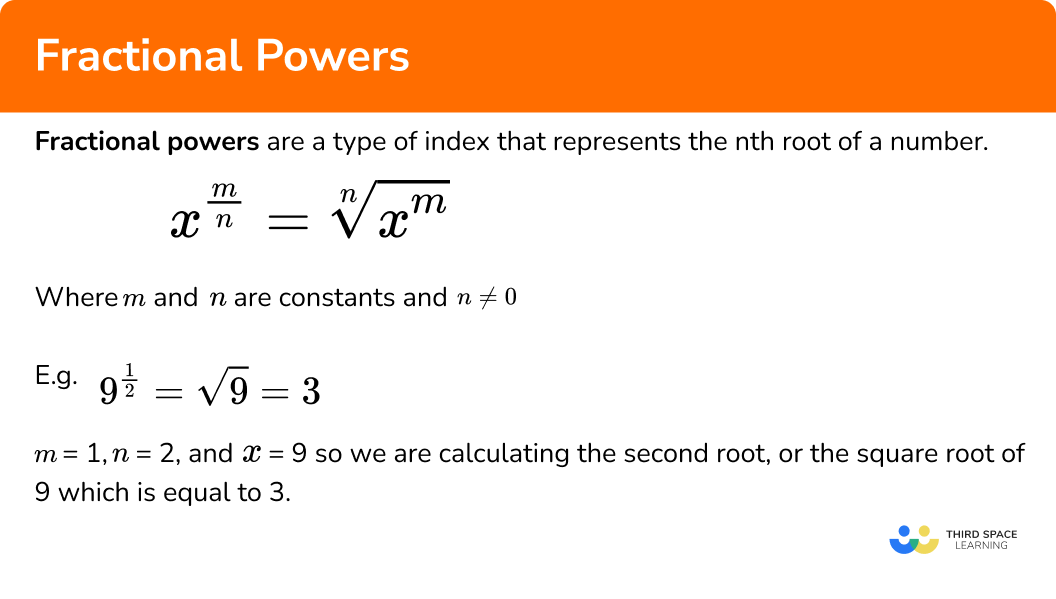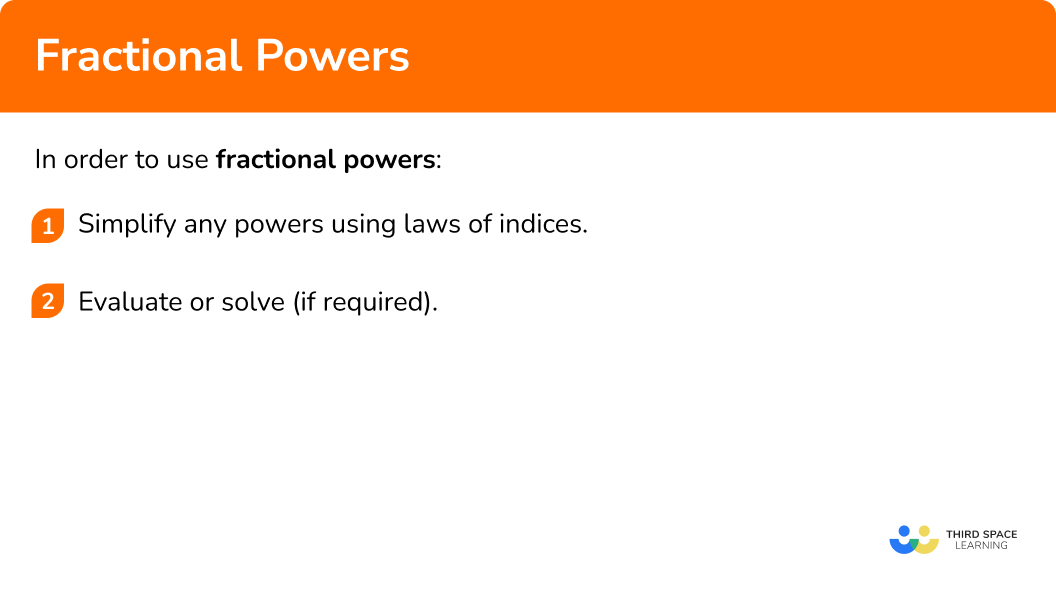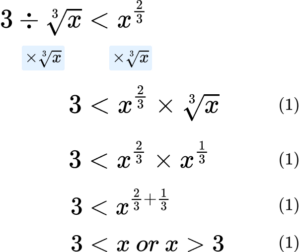GCSE Maths Number Powers And Roots

Fractional Powers

# Fractional Powers

Here we will learn about fractional powers, including what they are, how to simplify and evaluate them, and how to combine them with other laws of exponents.

There are also powers and roots worksheets based on Edexcel, AQA and OCR exam questions, along with further guidance on where to go next if you’re still stuck.

## What are fractional powers?

Fractional powers are a type of index that represents the nth root of a number.

In general, the rule for fractional exponents is x^{\frac{m}{n}}=\sqrt[n]{x^{m}} .

Where m and n are constants and n 0 .

For example,

9^{\frac{1}{2}}=\sqrt{9}=3

m=1, n=2, and x=9 , so we are calculating the second root, or the square root of 9 which is equal to 3 .

### What are fractional powers?## How to use fractional powers

In order to use fractional powers:

1. Simplify any powers using laws of indices.
2. Evaluate or solve (if required).

### How to use fractional powers### Related lessons onpowers and roots

Fractional powers is part of our series of lessons to support revision on powers and roots. You may find it helpful to start with the main powers and roots lesson for a summary of what to expect, or use the step by step guides below for further detail on individual topics. Other lessons in this series include:

## Fractional powers examples

### Example 1: simplify and evaluate

Simplify and evaluate the expression

9^{\frac{1}{2}} \times\ 9 .

1. Simplify any powers using laws of indices.

As x^{a} \times x^{b}=x^{a+b} , and 9=9^{1} , we have

9^{\frac{1}{2}}=9^{\frac{1}{2}+1}=9^{1.5}=9^{\frac{3}{2}} .

2Evaluate or solve (if required).

Evaluating the expression, we get

9^{\frac{3}{2}}=(\sqrt{9})^{3}=3^{3}=27 .

So 9^{\frac{1}{2}}\times\ {9}=27 .

### Example 2: algebraic

Write the following expression in its simplest form

(xy)^{a}\div\ {(x)^\frac{a}{2}} .

Simplify any powers using laws of indices.

Evaluate or solve (if required).

### Example 3: inequalities

Solve the inequality

64^{\frac{1}{3}}\geq x^2 .

Simplify any powers using laws of indices.

Evaluate or solve (if required).

### Example 4: negative fractional exponents

Evaluate the following expression,

216^{-\frac{2}{3}} .

Simplify any powers using laws of indices.

Evaluate or solve (if required).

### Example 5: fractional base number

Simplify fully,

(2\frac{113}{256})^{\frac{1}{4}} .

Simplify any powers using laws of indices.

Evaluate or solve (if required).

### Example 6: expressing a power with a different base

Simplify fully,

(8^{-10})^{\frac{1}{5}} .

Simplify any powers using laws of indices.

Evaluate or solve (if required).

### Common misconceptions

• Fractional power and fraction of amounts

A common error is to mistake a fractional power with multiplying by a fraction.

For example,

27^{\frac{1}{3}}=27\times{\frac{1}{3}}=9 is incorrect.

This is because the denominator of the fraction is the nth root of the base.

27^{\frac{1}{3}}=\sqrt{27}=3 .

• BIDMAS

When raising a base to a power, the power is associated with a variable or an expression.

For example, let’s look at  3x^{-2} .

Using BIDMAS we can see that x is being raised to the power of -2 , and then is multiplied by 3 to get the answer \frac{3}{x^{2}}

However a common error is to calculate 3x raised to the power of -2 , giving the answer \frac{1}{9x^{2}} which is incorrect. If this was the case, the question would be written as (3x)^{-2}.

• Incorrect application of the exponent

A similar circumstance is applied to fractions that are raised to a power.

For example, let’s look at (\frac{5}{2})^{-3} .

The correct application of the laws of indices would give a correct answer of  (\frac{5}{2})^{-3}=(\frac{2}{5})^{3}=\frac{8}{125} .

However a common error would be to apply the -3 only to the numerator, leaving the denominator unchanged. This would give the incorrect answer of (\frac{5}{2})^{-3}=\frac{1}{125}\times\frac{1}{2}=\frac{1}{250} .

### Practice fractional powers questions

1. Evaluate

4^{\frac{1}{2}}\times\ {4^{\frac{3}{2}}} .

4^{\frac{3}{4}}128164^{\frac{1}{2}}\times\ {4^{\frac{3}{2}}}=4^{\frac{1}{2}+\frac{3}{2}}=4^{\frac{4}{2}}=4^{2}=16

2. Simplify fully,

(x^{a}y)^{\frac{1}{a}} .

\frac{x^{a}}{y^{a}}(xy)^{a}xy^{\frac{1}{a}}\frac{1}{ax^{a}y}(x^{a}y)^{\frac{1}{a}}=x^{a\times{\frac{1}{a}}}\times\ {y^{\frac{1}{a}}}=x\times\ {y^{\frac{1}{a}}}=xy^{\frac{1}{a}}

3. State the range of values for the following inequality,

8^{\frac{2}{3}}<\frac{x}{5} .

x>20x<20x>30x<308^{\frac{2}{3}}=(\sqrt{8})^{2}=2^{2}=4

4<\frac{x}{5}

20<x so x>20

4. Evaluate

625^{-\frac{3}{4}} .

-125125\frac{1}{125}-\frac{1}{125}625^{-\frac{3}{4}}=\frac{1}{625^{\frac{3}{4}}}=\frac{1}{(\sqrt{625})^{3}}=\frac{1}{5^{3}}=\frac{1}{125}

5. Evaluate

(\frac{1024}{32})^{\frac{1}{5}} .

\frac{1}{8}26.4160(\frac{1024}{32})^{\frac{1}{5}}=\frac{\sqrt{1024}}{\sqrt{32}}=\frac{4}{2}=2

6. Simplify the expression below. Write your answer in the form 3^{n}.

(9^{-3})^{\frac{1}{2}}

-\frac{3}{2}3^{-3}\frac{1}{27}27(9^{-3})^{\frac{1}{2}}=(9^{\frac{1}{2}})^{-3}=3^{-3}

### Fractional powers GCSE questions

1. Solve the inequality 3\div \sqrt{x}<x^{\frac{2}{3}} .

(4 marks)2. (a) Evaluate 16^{-1\frac{1}{2}} .

(b) Simplify a^{\frac{1}{2}}\times{a^{\frac{1}{2}}} .

(5 marks)

(a)

-1\frac{1}{2}=-\frac{3}{2}

(1)

\sqrt{16}=4

(1)

4^{-3}=\frac{1}{4^{3}}=\frac{1}{64}

(1)

(b)

a^{\frac{1}{2}}\times{a^{\frac{1}{2}}}=a^{\frac{1}{2}+\frac{1}{2}}

(1)

a^{1}

(1)

3. Express the following as a single power of 2 ,

4^{4}\times{\sqrt{8}} .

(6 marks)

4^{4}=(2^{2})^{4}

(1)

(2^{2})^{4}=2^{2\times 4}=2^{8}

(1)

\sqrt{8}=8^{\frac{1}{2}}=(2^{3})^{\frac{1}{2}}

(1)

2^{8}\times{2^{\frac{3}{2}}}

(1)

2^{8+\frac{3}{2}}

(1)

=2^{\frac{19}{2}}=2^{9\frac{1}{2}}

(1)

## Learning checklist

You have now learned how to:

• Consolidate your numerical and mathematical capability from key stage 3 and extend your understanding of the number system to fractional indices
• Calculate with roots, and fractional indices

## Still stuck?

Prepare your KS4 students for maths GCSEs success with Third Space Learning. Weekly online one to one GCSE maths revision lessons delivered by expert maths tutors.

Find out more about our GCSE maths tuition programme.